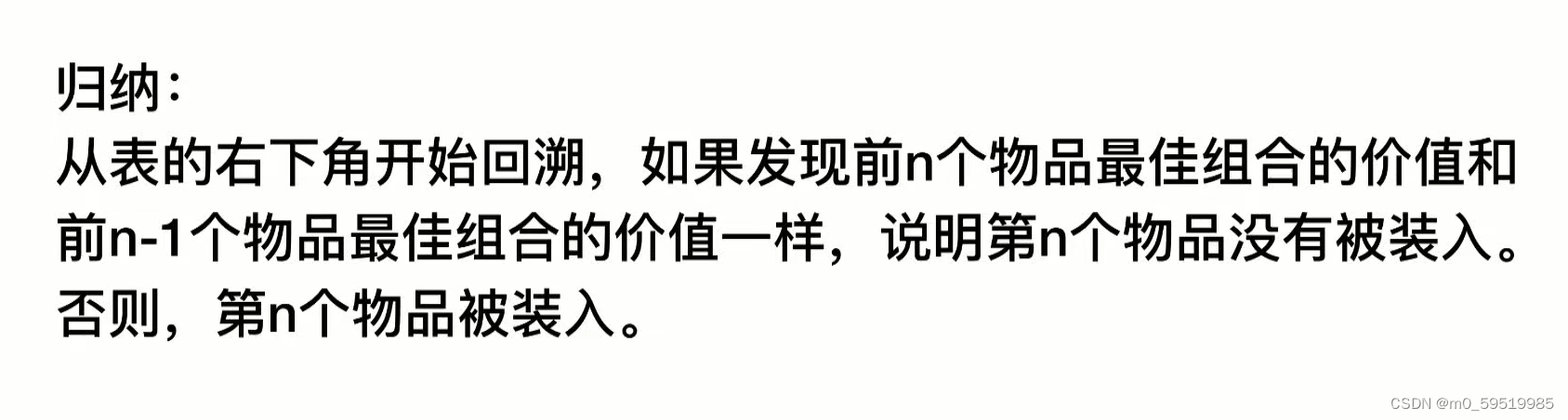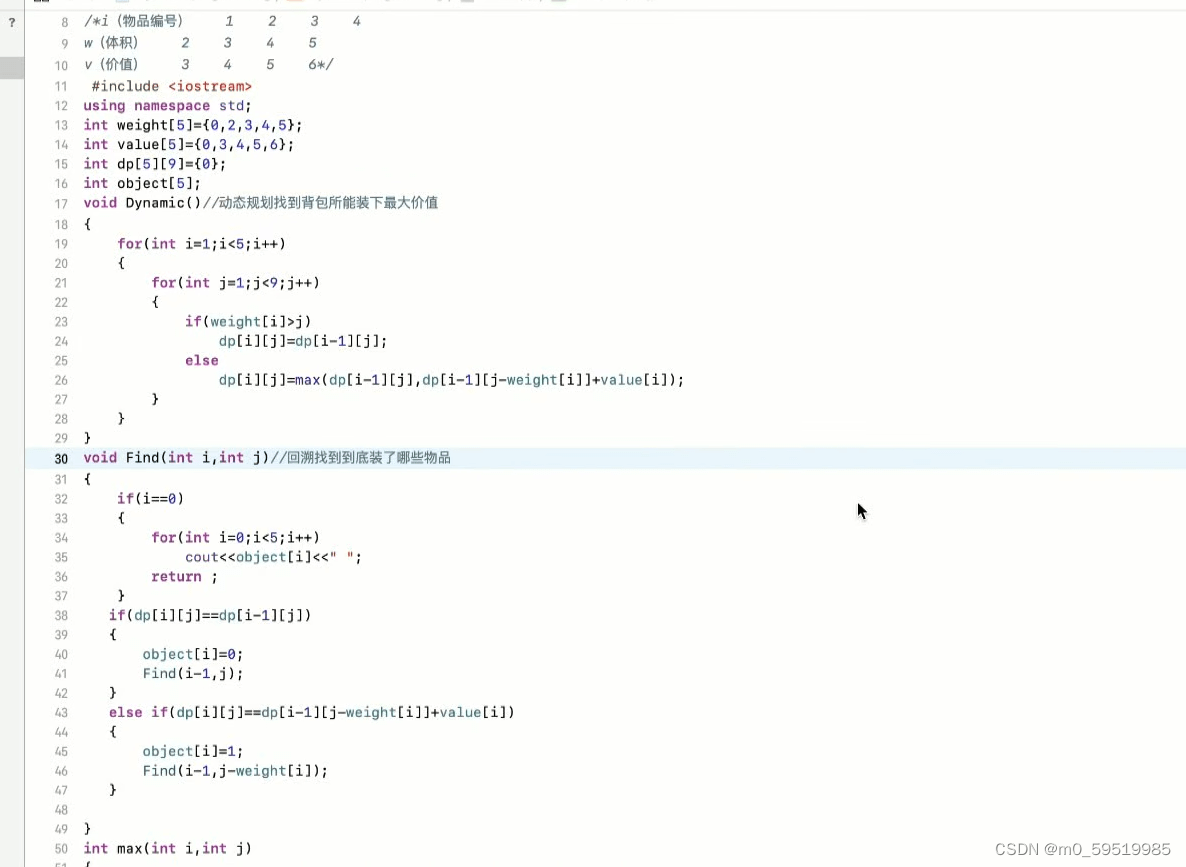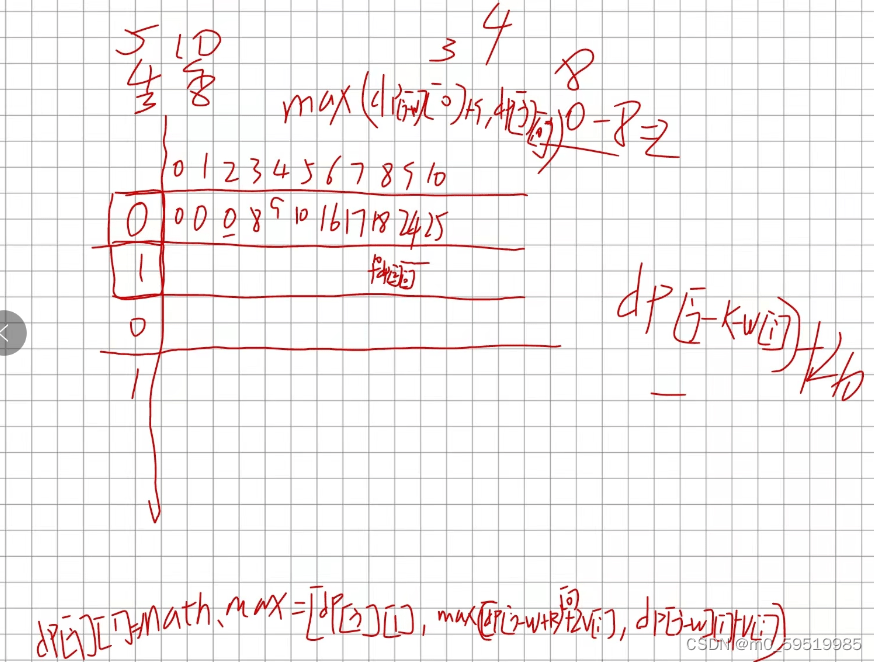# Dynamic programming topic (continued tomorrow)

### Description of topic

Blue is playing a game on a grid with nn rows and mm columns.

At the beginning, Xiaolan is standing in the upper left corner of the grid, which is row 11 and column 11.

Xiaolan can walk on the grid chart. When walking, if he is currently at row rr and column cc, he cannot go to a row with a row number smaller than rr, nor can he go to a column with a column number smaller than cc. At the same time, the straight-line distance he walks in one step does not exceed 33.

For example, if Xiaolan is currently at line 33, column 55, he can go to line 33, column 66, line 33, column 77, line 33, column 88, line 44, column 55, line 44 One of line 66, line 44, column 77, line 55, column 55, line 55, column 66, line 66, column 55.

Xiaolan will eventually go to the nnth row and the mmth column.

In the picture, some positions have rewards, which can be obtained by walking up, and some positions have punishments, which must be accepted by walking up. The reward and punishment are finally abstracted into a weight, the reward is positive, and the penalty is negative.

Xiaolan hopes that after going from the 11th row and the 11th column to the nnth row and the mmth column, the total weight sum is the largest. What is the maximum?

### Enter description

The first line of input contains two integers n,mn,m denoting the size of the graph.

The next nn lines, each with mm integers, represent the weight of each point in the grid.

Among them, 1≤n≤100, ?104≤weight≤1041≤n≤100, ?104≤weight≤104.

### output description

Output an integer representing the maximum sum of weights.

Example 1

enter

```3 5
-4 -5 -10 -3 1
7 5 -9 3 -10
10 -2 6 -10 -4
```

output

```15
```

### Run limit

• Maximum running time: 1s
• Maximum running memory: 128M

accomplish:

```import java.util.Scanner;
public class jump {
public static void main(String[] args) {
Scanner scan = new Scanner(System.in);
int n=scan.nextInt();
int m = scan. nextInt();
int[][] arr=new int[n][m];
for(int i=0;i<n;i ++ ) {
for(int j=0;j<m;j++) {
arr[i][j]=scan.nextInt();
}
}
scan. close();
int[][] b=new int[n][m];
b=arr;
for(int i=0;i<n;i ++ ) {
for(int j=0;j<m;j++) {
int c=3;
while(c>0) {
if(i-c>=0) {
b[i][j]=Math.max(b[i][j], b[i-c][j] + arr[i][j]);
}
if(j-c>=0) {
b[i][j]=Math.max(b[i][j], b[i][j-c] + arr[i][j]);
}
c--;
}
}
}
System.out.println(b[n-1][m-1]);
}
}
```

Dynamic programming to find the longest subsequence

### Description of topic

The organisms on planet L are composed of proteoids, each of which is composed of a class of substances called cyanopeptides that are connected end to end into a long chain and then folded.

Biologist Joe is researching protein on the planet L. She got the cyanopeptide sequences of two proteoids, and wanted to analyze the similarity of the two cyanopeptides through the common characteristics of the two cyanopeptide sequences.

Specifically, a cyanopeptide can be represented by 11 to 55 English letters, where the first letter is uppercase and the following letters are lowercase. A proteocyanin cyanopeptide sequence can be spliced with the sequence of cyanopeptide representation.

In a cyanopeptide sequence, if some positions are selected, the cyanopeptides in these positions are taken out, and arranged according to their positions in the original sequence, it is called a subsequence of the cyanopeptide. The subsequences of cyanopeptides are not necessarily continuous in the original sequence, and there may be some unremoved cyanopeptides in the middle.

If a subsequence that can be extracted from the first cyanopeptide sequence is equal to a certain subsequence extracted from the second cyanopeptide sequence, it is called a public cyanopeptide subsequence.

Given two cyanopeptide sequences, find the length of their longest common cyanopeptide subsequence.

### Enter a description

Enter two lines, each containing a string representing a cyanopeptide sequence. There are no separator characters such as spaces in the middle of the string.

Among them, the length of both strings does not exceed 10001000.

### Output description

Output an integer representing the length of the longest public blue peptide subsequence.

### Sample input and output

example

enter

```Lan Qiao Bei
Lan Tai Xiao Qiao
```

output

```2
```

### Run limit

• Maximum running time: 1s
• Maximum running memory: 128M
```import java.util.*;
public class lantai {
public static void main(String[] args) {
Scanner scan = new Scanner(System.in);
String str1 = scan. nextLine();
String str2 = scan. nextLine();
scan. close();
int[] flag1=new int[str1. length()];
int[] flag2=new int[str2. length()];
int c=0;
int m=0,n=0;
for(int i=0;i<str1. length();i ++ ) {
if(str1.charAt(i)>='A' & amp; & amp;str1.charAt(i)<='Z') {
flag1[c]=i;
c++;
m++;
}
}
flag1[c]=str1. length();
c=0;
for(int i=0;i<str2. length();i ++ ) {
if(str2.charAt(i)>='A' & amp; & amp;str2.charAt(i)<='Z') {
flag2[c]=i;
c++;
n + + ;
}
}
flag2[c]=str2. length();
int[][] dp=new int[m + 1][n + 1];
for(int i=0;i<m;i ++ ) {
for(int j=0;j<n;j++) {
// System.out.println(str1.substring(flag1[i], flag1[i + 1]) + " " + str2.substring(flag2[j], flag2[j + 1]));
if(str1.substring(flag1[i], flag1[i + 1]).equals(str2.substring(flag2[j], flag2[j+1]))) {
dp[i + 1][j + 1]=Math.max(dp[i + 1][j + 1], dp[i][j] + 1);
}else{
dp[i + 1][j + 1]=Math.max(dp[i][j + 1], dp[i + 1][j]);
}
}
}

System.out.println(dp[m][n]);
}
}
```

In the above two cases, a segmentation fault occurs,

```import java.util.Scanner;
// 1: no package required
// 2: The class name must be Main, which cannot be modified

public class Main {
public static void main(String[] args) {
Scanner scan = new Scanner(System.in);
String str1 = scan. nextLine();
String str2 = scan. nextLine();
scan. close();
int[] flag1=new int[str1. length() + 1];
int[] flag2=new int[str2. length() + 1];
int c=0;
int m=0,n=0;
for(int i=0;i<str1. length();i ++ ) {
if(str1.charAt(i)>='A' & amp; & amp;str1.charAt(i)<='Z') {
flag1[c]=i;
c++;
m++;
}
}
flag1[c]=str1. length();
c=0;
for(int i=0;i<str2. length();i ++ ) {
if(str2.charAt(i)>='A' & amp; & amp;str2.charAt(i)<='Z') {
flag2[c]=i;
c++;
n + + ;
}
}
flag2[c]=str2. length();
int[][] dp=new int[m + 1][n + 1];
for(int i=1;i<=m;i ++ ) {
for(int j=1;j<=n;j++) {
// System.out.println(str1.substring(flag1[i-1], flag1[i]) + " " + str2.substring(flag2[j-1], flag2[j]));
if(str1.substring(flag1[i-1], flag1[i]).equals(str2.substring(flag2[j-1], flag2[j]))) {
dp[i][j]=Math.max(dp[i][j], dp[i-1][j-1] + 1);
}else{
dp[i][j]=Math.max(dp[i-1][j], dp[i][j-1]);
}
}
}

System.out.println(dp[m][n]);
}
}```

Make up the number of buns:

### Description of topic

Xiao Ming eats breakfast at a bun shop almost every morning. He found that this steamed bun shop has NN kinds of steamers, of which the ii kind of steamer can just hold AiAi? steamed buns. Each steamer has so many cages that it can be thought of as infinite cages.

Whenever a customer wants to buy XX buns, the uncle who sells buns will quickly select a number of steamed buns, so that there are exactly XX steamed buns in these cages. For example, there are 3 kinds of steamers, which can hold 3, 4 and 5 buns respectively. When a customer wants to buy 11 steamed buns, the uncle will choose 2 cages with 3 buns and 1 cage with 5 buns (may also choose 1 cage with 3 buns and 2 cages with 4 buns).

Of course, sometimes Uncle Baozi can’t make up the quantity that customers want to buy anyway. For example, there are 3 kinds of steamers, which can hold 4, 5 and 6 buns respectively. And when the customer wanted to buy 7 steamed buns, the uncle couldn’t get them together.

Xiao Ming wants to know how many numbers there are that Uncle Baozi can’t make up.

### Enter a description

The first line contains an integer NN (1≤N≤1001≤N≤100).

Each of the following N lines contains an integer AiAi? (1≤Ai≤1001≤Ai?≤100).

### Output description

An integer representing the answer. If there are infinitely many numbers that cannot be made up, output INF.

### Sample input and output

Example 1

enter

```2
4
5
```

output

```6
```

Sample description

Numbers that cannot be made include: 1, 2, 3, 6, 7, 11.

Example 2

enter

```2
4
6
```

output

```INF
```

Sample description

All odd numbers cannot be made up, so there are infinitely many

### Run limit

• Maximum running time: 1s
• Maximum running memory: 256M
```import java.util.*;
public class baozicoushu {
public static int gcd(int a,int b) {
if(b==0) {
return a;
} else {
return gcd(b,a%b);
}
}
public static void main(String[] args) {
Scanner scan = new Scanner(System.in);
int n=scan.nextInt();
int[] a=new int[n + 1];
int s;
for(int i=1;i<=n;i ++ ) {
a[i]=scan.nextInt();
}
scan. close();
s=a;
for(int i=1;i<=n;i ++ ) {
s=gcd(s,a[i]); // Find the greatest common divisor (the greatest common divisor of the number of steamed buns entered)
}
if(s!=1) { //These numbers contain the greatest common divisor and are not mutually prime, such as 3, 6, 9, the numbers that cannot be formed by these numbers are infinite
System.out.println("INF");
}else{
boolean[] f=new boolean;
f=true;
for(int i=1;i<=n;i ++ ) {
for(int j=0;j<10001;j ++ ) { //Mark the array and look for numbers that cannot be made
if(f[j] & amp; & amp;(j + a[i])<10001) {
f[j + a[i]]=true;
}
}
}
int sum=0;
for(int i=1;i<10001;i ++ ) {
if(!f[i]) {
sum + + ;
}
}
System.out.println(sum);
}
\t\t
}
}
```

01 backpack problem##Trackback:```import java.util.*;
public class ZeroAndOne {
public static void main(String[] args) {
int[][] dp=new int;
int[] w= {0,2,3,4,5}; //The capacity corresponding to the item
int[] v= {0,3,4,5,8}; //The value corresponding to the item
for(int i=1;i<5;i ++ ) {
for(int j=1;j<9;j ++ ) { //j represents the backpack capacity
if(w[i]>j) {
dp[i][j]=dp[i-1][j];
} else {
dp[i][j]=Math.max(dp[i-1][j],dp[i-1][j-w[i]] + v[i]);
} //Do not steal the i-1th item Steal
}
}
System.out.print(dp);
}
}
```

Backpacks and Magic

### Problem Description

There are NN items in front of Xiaolan, among which the weight of item ii is WiWi?, and the value is ViVi?. She also has a backpack with a maximum load capacity of MM.

Xiaolan wants to know what is the maximum value of items she can hold within the weighing range of her backpack?

It is particularly worth mentioning that Xiaolan can use a spell (used once in total) to increase the weight of an item by KK, and at the same time the value is doubled. (Of course, Xiaolan can also not use magic)

### Input format

The first line contains 3 integers N, MN, M and KK .

The following NN lines, each with two integers WiWi? and ViVi?.

### sample input

```3 10 3
5 10
4 9
3 8
```

```26
```

### Sample Description

Select the second and third items while using magic on the second item.

### Evaluation use case scale and agreement

For 300% of the data, 1≤N,M,K≤1001≤N,M,K≤100.

For 100 0% of the data, 1≤N≤2000,1≤M,K≤10000,0≤Wi,Vi≤100001≤N≤2000,1≤M,K≤10000,0≤Wi?,Vi?≤ 10000.

### Run limit

Language Maximum runtime Maximum memory
C++ 1s 256M
C 1s 256M
Java 3s 256M
Python3 5s 256M

State transition equation:

If you do not use magic, that is, when j=0, it is the same as the template of the 01 backpack,
dp[j] = Math.max(dp[j],dp[j-w[i]] + v[i])
If magic is used, (to ensure that the size of j meets the conditions)
There are two cases, this item uses magic or one of the items used magic before
1. If the item uses magic this time, that is, Math.max(dp[j-w[i]-k] + 2*v[i]
2. If one of the previous items uses magic, that is, dp[j-w[i]] + v[i]
Together it is dp[j] = Math.max(dp[j],Math.max(dp[j-w[i]-k] + 2*v[i],dp[j-w [i]] + v[i]));```import java.util.*;
public class PackageAndMagin {
public static void main(String[] args) {
Scanner scan = new Scanner(System.in);
int n = scan. nextInt();
int m = scan. nextInt();
int k = scan. nextInt();
int[] w = new int[n + 1];
int[] v = new int[n + 1];
w=0;
v=0;
for(int i=1;i<=n;i ++ ) {
w[i]=scan.nextInt();
v[i]=scan.nextInt();
}
scan. close();
// int[][] dp=new int[n + 1][m + 1];
// for(int i=1;i<n + 1;i + + ) {
// for(int j=1;j<m + 1;j + + ) {
// if(j<w[i]) {
// dp[i][j]=dp[i-1][j];
// } else {
// System.out.println(i + " " + j);
// dp[i][j]=Math.max(dp[i-1][j], dp[i-1][j-w[i]] + v[i]);
// System.out.println(i + " " + j);
// }
// }
// }
int[][] dp=new int[m + 1];
for(int i=1;i<n + 1;i + + ) {
for(int j=m;j>=w[i];j--) {
if(j>=k + w[i]) {//Use the potion or use the potion before
dp[j]=Math.max(dp[j],dp[j-w[i]] + v[i]);
//Optimal combination without using potion Do not install the optimal combination of the current capacity of this item, install the optimal combination of the current item
dp[j]=Math.max(dp[j], Math.max(dp[j-w[i]-k] + 2*v[i], dp[j-w[i] ] + v[i]));
// The optimal combination of using the potion before but not loading the item this time, the optimal combination of using the potion and loading this item this time, this time not using the potion but using the optimal combination of the potion before
} else {
dp[j]=Math.max(dp[j], dp[j-w[i]] + v[i]);
}
}
}
System.out.println(Math.max(dp[m], dp[m]));
}
}
```

The knowledge points of the article match the official knowledge files, and you can further learn relevant knowledge algorithm skill treehome page overview 41947 people are learning